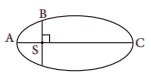# UNIT VI: Gravitation - Online Test

Q1. The linear momentum and position vector of the planet is perpendicular to each other at
Explaination / Solution:
No Explaination.

Q2. If the masses of the Earth and Sun suddenly double, the gravitational force between them will
Explaination / Solution:
No Explaination.

Q3. A planet moving along an elliptical orbit is closest to the Sun at distance r1 and farthest away at a distance of r2. If v1 and v2 are linear speeds at these points respectively. Then the ratio V1/V2 is
Explaination / Solution:
No Explaination.

Q4. The time period of a satellite orbiting Earth in a cirular orbit is independent of
Explaination / Solution:
No Explaination.

Q5. If the distance between the Earth and Sun were to be doubled from its present value, the number of days in a year would be
Explaination / Solution:
No Explaination.

Q6. According to Kepler’s second law, the radial vector to a planet from the Sun sweeps out equal areas in equal intervals of time. This law is a consequence of
Explaination / Solution:
No Explaination.

Q7. The gravitational potential energy of the Moon with respect to Earth is
Explaination / Solution:
No Explaination.

Q8. The kinetic energies of a planet in an elliptical orbit about the Sun, at positions A, B and C are KA, KB and KC respectively. AC is the major axis and SB is perpendicular to AC at the position of the Sun S as shown in the figure.Then
Explaination / Solution:
No Explaination.

Q9. The work done by the Sun’s gravitational force on the Earth is
Explaination / Solution:
No Explaination.

Q10. If the mass and radius of the Earth are both doubled, then the acceleration due to gravity g'
Explaination / Solution:
No Explaination.